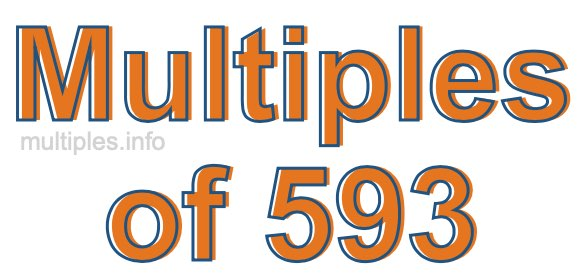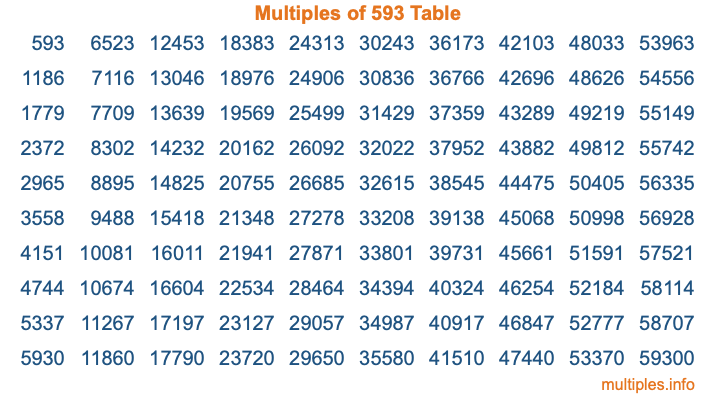Multiples of 593Welcome to the Multiples of 593 page. Here we will first teach you everything you will ever need to know about the multiples of 593, and then give you a study guide summary of everything we taught you to make sure you remember it all. Use this page to look up facts and learn information about the multiples of 593. This page will make you a multiples of five hundred ninety-three expert!

Definition of Multiples of 593
Multiples of 593 are all the numbers that when divided by 593 equal an integer. Each of the multiples of 593 are called a multiple. A multiple of 593 is created by multiplying 593 by an integer.

Therefore, to create a list of multiples of 593, you start with 1 multiplied by 593, then 2 multiplied by 593, then 3 multiplied by 593, and so on for as long as you want. Thus, the list of the first five multiples of 593 is 593, 1186, 1779, 2372, and 2965. To see a larger list of multiples of 593, see the printable image of Multiples of 593 further down on this page. We also have a category where you can choose any nth multiple of 593.

Multiples of 593 Checker
The Multiples of 593 Checker below checks to see if any number of your choice is a multiple of 593. In other words, it checks to see if there is any number (integer) that when multiplied by 593 will equal your number. To do that, we divide your number by 593. If the the quotient is an integer, then your number is a multiple of 593.

Is  a multiple of 593?

Least Common Multiple of 593 and ...
A Least Common Multiple (LCM) is the lowest multiple that two or more numbers have in common. This is also called the smallest common multiple or lowest common multiple and is useful to know when you are adding our subtracting fractions. Enter one or more numbers below (593 is already entered) to find the LCM.

Check out our LCM Calculator if you need more details about the Least Common Multiple or if you need the LCM for different numbers for adding and subtraction fractions.

nth Multiple of 593
As we stated above, 593 is the first multiple of 593, 1186 is the second multiple of 593, 1779 is the third multiple of 593, and so on. Enter a number below to find the nth multiple of 593.

th multiple of 593

Multiples of 593 vs Factors of 593
593 is a multiple of 593 and a factor of 593, but that is where the similarities end. All postive multiples of 593 are 593 or greater than 593. All positive factors of 593 are 593 or less than 593.

Below is the beginning list of multiples of 593 and the factors of 593 so you can compare:

Multiples of 593: 593, 1186, 1779, 2372, 2965, etc.

Factors of 593: 1, 593

As you can see, the multiples of 593 are all the numbers that you can divide by 593 to get a whole number. The factors of 593, on the other hand, are all the whole numbers that you can multiply by another whole number to get 593.

It's also interesting to note that if a number (x) is a factor of 593, then 593 will also be a multiple of that number (x).

Multiples of 593 vs Divisors of 593
The divisors of 593 are all the integers that 593 can be divided by evenly. Below is a list of the divisors of 593.

Divisors of 593: 1, 593

The interesting thing to note here is that if you take any multiple of 593 and divide it by a divisor of 593, you will see that the quotient is an integer.

Multiples of 593 Table
Below is an image of the first 100 multiples of 593 in a table. The table is in chronological order, column by column. The first column has the first ten multiples of 593, the second column has the next ten multiples of 593, and so on.The Multiples of 593 Table is also referred to as the 593 Times Table or Times Table of 593. You are welcome to print out our table for your studies.

Negative Multiples of 593
Although not often discussed or needed in math, it is worth mentioning that you can make a list of negative multiples of 593 by multiplying 593 by -1, then by -2, then by -3, and so on, to get the following list of negative multiples of 593:

-593, -1186, -1779, -2372, -2965, etc.

Multiples of 593 Summary
Below is a summary of important Multiples of 593 facts that we have discussed on this page. To retain the knowledge on this page, we recommend that you read through the summary and explain to yourself or a study partner why they hold true.

There are an infinite number of multiples of 593.

A multiple of 593 divided by 593 will equal a whole number.

593 divided by a factor of 593 equals a divisor of 593.

The nth multiple of 593 is n times 593.

The largest factor of 593 is equal to the first positive multiple of 593.

593 is a multiple of every factor of 593.

593 is a multiple of 593.

A multiple of 593 divided by a divisor of 593 equals an integer.

593 divided by a divisor of 593 equals a factor of 593.

Any integer times 593 will equal a multiple of 593.

Multiples of a Number
Here you can get the multiples of another number, all with the same attention to detail as we did for multiples of 593 on this page.

Multiples of
Multiples of 594
Did you find our page about multiples of five hundred ninety-three educational? Do you want more knowledge? Check out the multiples of the next number on our list!

Copyright  |   Privacy Policy  |   Disclaimer  |   Contact